# Operators In JavaScript

in STEMGeekslast year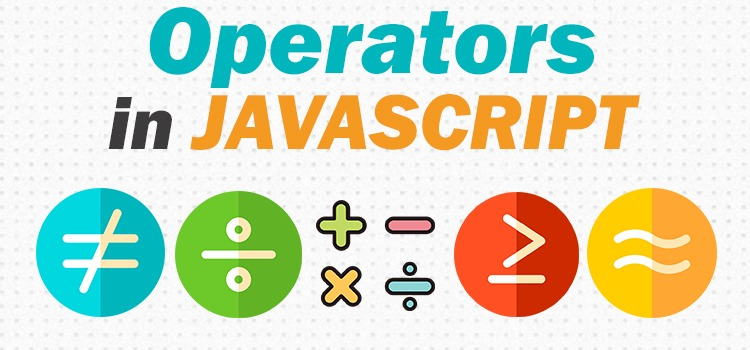Image Source

Operators are an important aspects of every programming language. They are used to perform certain operations on the single or multiple operands to produe the desired results. In JavaScript, we have following main types of operator:

### 1. Assignment Operator

Assignment operator is used to assign a value to the variable in JavaScript. The most common example would be `x=5` where left operand i.e. x is assigned a value of right operand which is 5. There are various types of assignment operator that falls under this category like `x+=5` also known as addition assignment operator which also means `x=6`. In the same way there is also multiplication assignment operator. For example: `x*=6`.

### 2. Arithmetic Operator

Arithmetic operator is used to perform certain arithmetic operations on the operands like addition, subtraction, multiplication, division. The following is a basic example demonstrating the same.

``````<!DOCTYPE html>
<html lang="en">
<meta charset="UTF-8">
<meta http-equiv="X-UA-Compatible" content="IE=edge">
<meta name="viewport" content="width=device-width, initial-scale=1.0">
<title>JavaScript</title>
<script>
var x=20;
var y=5;
var z = x*y;
var p = x/y;
document.write(z)

document.write("<br>")

document.write(p)

</script>

<body>

</body>
</html>
``````

The output is: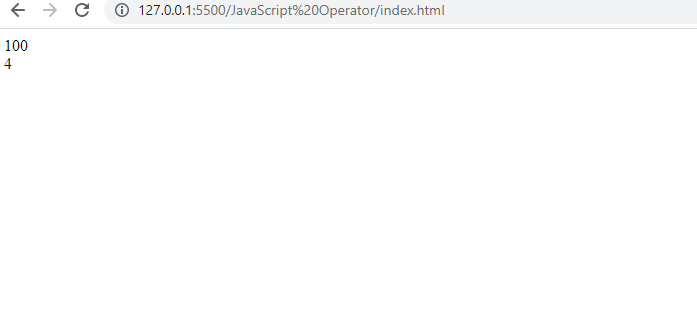### 3. Comparison Operator

Comparison operators are used to compare two operands and they return a Boolean value. For example in the console bar of your browser window you can try examining this code: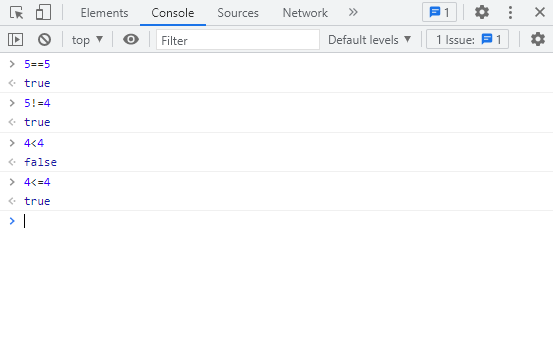### 4. Logical Operator

These operators are used to perform logical operations on the operands and they also return a Boolean value i.e. True or False. The following example demonstrates three basic types of logical operation: AND, OR and NOT.

``````<!DOCTYPE html>
<html lang="en">
<meta charset="UTF-8">
<meta http-equiv="X-UA-Compatible" content="IE=edge">
<meta name="viewport" content="width=device-width, initial-scale=1.0">
<title>JavaScript</title>
<script>
var x=20;
var y=5;
var z = x*y;

document.write(x>10 && y>6)

document.write("<br>")

document.write(x>25 || y<10)

document.write("<br>")

document.write(x!=21)

</script>

<body>

</body>
</html>
``````

It shows the following output: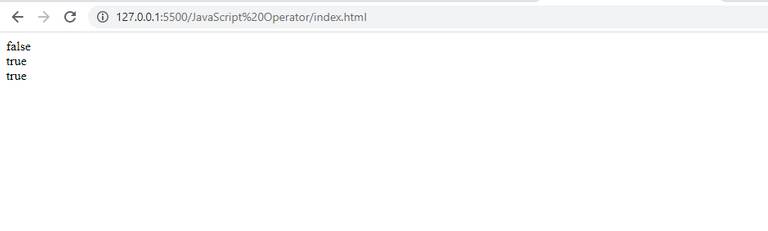We assign x the value of 20 and y the value of 5. `document.write(x>10 && y>6)` will return false since (20>10 AND 5>6) implies (T AND F) which gives the value of F. You need to know basic logic gate value to understand this. The same goes for the "OR" operation where `document.write(x>25 || y<10)` will return (20>25 || 5<10) which means (F OR T) and the OR operation of this value will return True.

### 5. String Operator

It is also known as the concatenation operator since it is used to concatenate two or more string values. For example the following code will join Hello and World and returns the output as expected.

``````<script>
var a = "Hello"
var b = "World"
var c = a + " " + b
document.write(c)

</script>
``````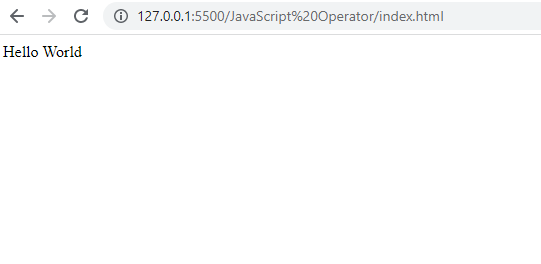### 6. Ternary Operator

This operator works like an if-else statement but offers the advantage of making your code concise, clear and short depending upon the condition. The syntax is:

``````condition ? expression1 : expression2
``````

A simple code to understand this one would be:

``````<script>
var a = 10;
document.write((a>=20)?"Greater than 20":"Smaller than 20");
</script>
``````

We first wrote our condition i.e. a>=20 followed by a question mark (?). After the question mark then we specify the statement we want to show. If condition is true the left statement is executed i.e. greater than 20 if not right statement i.e. smaller than 20. In our case the right statement is to be executed. You can see the same in the browser window.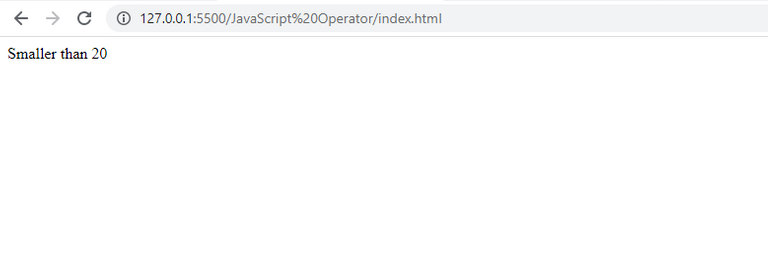There are many other types of operators but these all cover the basic you need to kickstart and you can learn while you progress along the way.